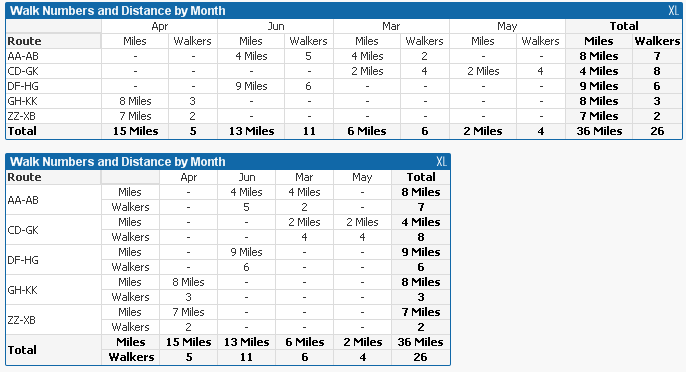# QlikView Layout & Visualizations

Discussion Board for collaboration on QlikView Layout & Visualizations.Partner

## Pivot Table SubTotals when a Dimension determines Expression Calculation

I am trying to build a Pivot Table with a single dimension on the left hand side, and Months of the Year along the top.

But then I have a floating table with a field called "ReportType" and this has two values: Number of People & Distance Walked

I add this field as a second dimension to the Pivot Table so that the two options appear on the left hand side after each value reported from the first dimension (in this example the first dimension is "route of walk" ). The Month is the 3rd dimension, and displayed along the top.

In the expression I check the ReportType value and either sum the people or sum the distance accordingly. So the Pivot Table shows two types of values against each "Route of Walk" i.e. Num of people per Month and Total Distance Walked per Month.

If I add partial sums to the Month Dimension, I get a nice total on the right hand side showing Total number of people and distance walked per route for all months. That is fine (although the unit of one total is a bit strange?)

But trying to display partial sums for the first dimension to give me total number of people and also distance walked per Month, for all routes, at the bottom of the table doesn't work. It sums the values for the first Report Type, but ignores the second?

I have attached a ZIP file containing some very basic data in EXCEL + a QVW which illustrates the problem.

Does anyone have any ideas how I could get this to behave as I want it to?

Thanks in advance if you do1 Solution

Accepted Solutions
HighlightedMVP & Luminary

## Re: Re: Pivot Table SubTotals when a Dimension determines Expression Calculation

The default way of doing this would be to have two separate expressions.  These would then show in either of these ways:Would either of those work for what you are after?

Code behind both tables attached.

Cheers,

Steve

4 Replies
HighlightedMVP & Luminary

## Re: Pivot Table SubTotals when a Dimension determines Expression Calculation

Hi there,

You need to do something different with the expression on the total row (as the report type will be both 1 and 2 at this point).  One way of identifying this is with the dimensionality function.  This will put both walkers and miles in the total field:

if(Dimensionality() = 0, Num(Sum(NumPeople),'#,##0') & ' / ' & Num(Sum(Distance),'#,##0 Miles'),

If(ReportType=1,

Num(Sum(Distance),'#,##0 Miles'),

Num(Sum(NumPeople),'#,##0')))

I tried getting it onto two rows (using CRLF instead of / ), but I couldn't get it to expand the total row without expanding all other cells as well.

Hope that points you in the right direction.

Steve

HighlightedPartner

## Re: Pivot Table SubTotals when a Dimension determines Expression Calculation

Hi Steve

Many thanks for your suggestion. It does work, and is at least a good step towards what I wanted.

It isn't exactly the requirement, and I'm wondering if I could get to where I want by changing the model / script, but it at least provides both totals, albeit in the same cell.

Cheers SteveHighlightedMVP & Luminary

## Re: Re: Pivot Table SubTotals when a Dimension determines Expression Calculation

The default way of doing this would be to have two separate expressions.  These would then show in either of these ways:Would either of those work for what you are after?

Code behind both tables attached.

Cheers,

Steve

HighlightedPartner

Thanks Steve